Courses

# 28 Year NEET Previous Year Questions: Some Basic Concepts Of Chemistry - 2

## 25 Questions MCQ Test Chemistry Class 11 | 28 Year NEET Previous Year Questions: Some Basic Concepts Of Chemistry - 2

Description
This mock test of 28 Year NEET Previous Year Questions: Some Basic Concepts Of Chemistry - 2 for NEET helps you for every NEET entrance exam. This contains 25 Multiple Choice Questions for NEET 28 Year NEET Previous Year Questions: Some Basic Concepts Of Chemistry - 2 (mcq) to study with solutions a complete question bank. The solved questions answers in this 28 Year NEET Previous Year Questions: Some Basic Concepts Of Chemistry - 2 quiz give you a good mix of easy questions and tough questions. NEET students definitely take this 28 Year NEET Previous Year Questions: Some Basic Concepts Of Chemistry - 2 exercise for a better result in the exam. You can find other 28 Year NEET Previous Year Questions: Some Basic Concepts Of Chemistry - 2 extra questions, long questions & short questions for NEET on EduRev as well by searching above.
QUESTION: 1

### At S.T.P. the density of CCl4 vapours in g/L will be nearest to: 

Solution:

1 mol CCl4 vapour = 12 + 4 × 35.5 = 154 g ≡ 22.4 L at STP

∴ Density =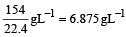QUESTION: 2

### One litre hard water contains 12.00 mg Mg2+.Mili-equivalents of washing soda required to remove its hardness is: 

Solution: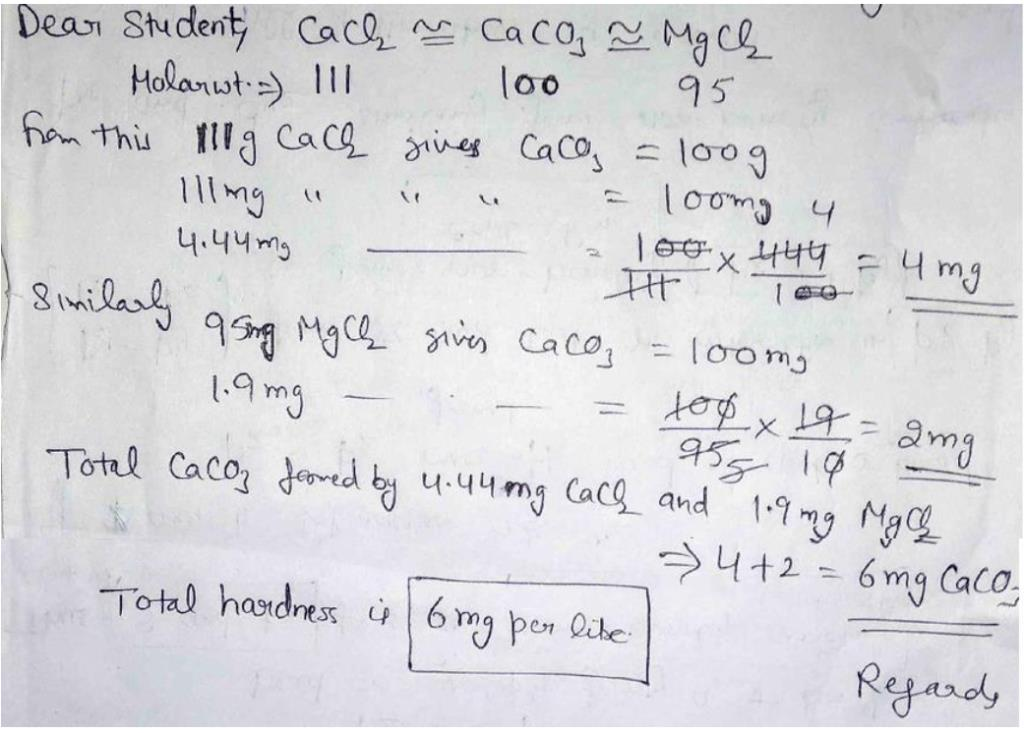QUESTION: 3

### 1 c.c. N2O at NTP contains :

Solution:

As we know, 22400 cc of N2O contain 6.02 x 1023 molecules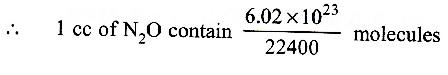Since in N2O molecule there are 3 atoms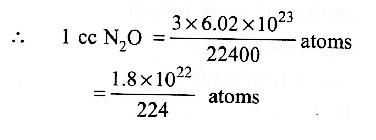No. of electrons in a molecule of N2O =  7 + 1 + 8 = 22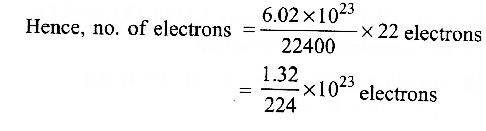QUESTION: 4

A metal oxide has the formula Z2O3. It can be reduced by hydrogen to give free metal and water. 0.1596 g of the metal oxide requires 6 mg of hydrogen for complete reduction. The atomic weight of the metal is 

Solution:

The reactioin may given as

Z2 O3 + 3H2 —→ 2Z + 3H2O
0.1596 g of Z2O3 react with H2
= 6 mg = 0.006 g
∴ 1 g of H2 react with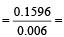26.6g of Z2O3

∴ Eq. wt. of Z2O3 = 26.6 (from the elefinition of eq. wt.) Eq. wt. of Z + Eq. wt. of O = E + 8 = 26.6

⇒  Eq. wt. of Z = 26.6 – 8 = 18.6 Valency of metal in Z2O3 = 3

Eq. wt.of metal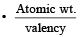∴ At. wt. of Z = 18.6 × 3 = 55.8

QUESTION: 5

Ratio of Cp and Cv of a gas ‘X’ is 1.4. The number of atoms of the gas ‘X’ present in 11.2 litres of it at NTP will be 

Solution:

Cp / Cv = 1.4 shows that the gas is diatomic. 22.4 litre at NTP ≡ 6.02 × 1023 molecules 11.2 L at NTP = 3.01 × 1023 molecules
= 3.01 × 1023 × 2 atoms = 6.02 × 1023 atoms

QUESTION: 6

What is the weight of oxygen required for the complete combustion of 2.8 kg of ethylene?

Solution:

C2H4 + 3 O2 —→ 2CO2 + 2H2O 28 kg    96 kg
∵ 28 kg of C2H4 undergo complete combustion  by = 96 kg of O2
∴ 2.8 kg of C2H4 undergo complete combustion by = 9.6 g of O2.

QUESTION: 7

The number of gram molecules of oxygen in 6.02 × 1024 CO molecules is 

Solution:

6.02 × 1023 molecules of CO =1mole of CO 6.02 × 1024 CO molecules = 10 moles CO = 10 g atoms of O = 5 g molecules of O2

QUESTION: 8

The number of oxygen atoms in 4.4 g of CO2 is

Solution:

4.4 g CO2 =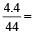0.1 mol CO2

(mol. wt. of CO2 = 44)
= 6 × 1022 molecules
= 2 × 6 × 1022 atoms of O

QUESTION: 9

Boron has two stable isotopes, 10B (19%) and 11B (81%). Average atomic weight for boron in the periodic table is 

Solution:

Average atomic mass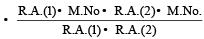Where R.A. = relative abundance
M.No = Mass number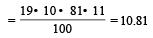QUESTION: 10

The molecular weight of O2 and SO2 are 32 and 64 respectively. At 15°C and 150 mm Hg pressure, one litre of O2 contains ‘N’ molecules. The number of molecules in two litres of SO2 under the same conditions of temperature and pressure will be : 

Solution:

A ccording to Avogadro's law "equal volumes of all gases contain equal numbers of molecules under similar conditions of temperature and pressure". Thus if 1 L of one gas contains N molecules, 2 L of any gas under the same conditions will contain 2N molecules.

QUESTION: 11

A 5 molar solution of H2SO4 is diluted from 1 litre to a volume of 10 litres, the normality of the solution will be : 

Solution:

5 MH2SO4 = 10 N H2SO4, (∵Basicity of H2SO4 = 2) N1V1 = N2V2,
10 × 1 = N2 × 10 or N2 = 1 N

QUESTION: 12

If NA is Avogadro’s number then number of valence electrons in 4.2g of nitride ions (N3–) is

Solution:

No of moles of nitride ion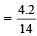= 0.3 mol 0.3 x NA  nitride ions.
Valence electrons = 8 × 0.3 NA = 2.4 NA (5 + 3 due to charge). One N3– ion contains 8 valence electrons.

QUESTION: 13

In the final answer of the expression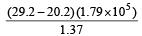the number of significant figures is : 

Solution:

On calculation we find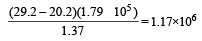As the least precise number contains 3 significant figures therefore answers should also contains 3 significant figures.

QUESTION: 14

The weight of one molecule of a compound C 60 H122 is 

Solution:

Molecular weight of C60H122 = (12 × 60) + 122 = 842.
Therefore weight of one molecule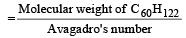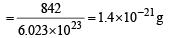QUESTION: 15

The percentage weight of Zn in white vitriol [ZnSO4.7H2O] is approximately equal to ( Zn = 65, S = 32, O = 16 and H = 1) 

Solution:

Molecular weight of ZnSO4 .7H 2O = 65 + 32 + (4 × 16) + 7(2 × 1 + 16) = 287.
∴ percentage mass of zinc (Zn)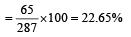QUESTION: 16

Liquid benzene (C6H6) burns in oxygen according to the equation 2C6H6(l ) + 15O2(g) —→ 12CO2(g)  6H2O(g) How many litres of O2 at STP are needed to complete the combustion of 39 g of liquid benzene?(Mol. wt. of O2 = 32, C6H6 = 78)  

Solution: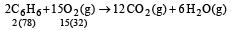156 gm of benzene required oxygen = 15 × 22.4 litre

∴ 1 gm of benzene required oxygen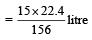∴ 39 gm of Benzene required oxygen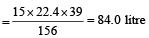QUESTION: 17

An  organic compound containing C, H and N gave the following analysis : C = 40% ; H = 13.33% ; N = 46.67%Its empirical formula would be 

Solution:

As the sum of the percentage of C, H & N is 100. Thus it does not contains O atom.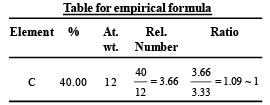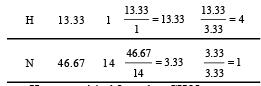Hence empirical formula = CH4N

QUESTION: 18

The number of significant figures for the three numbers 161 cm, 0.161 cm, 0.0161 cm are

Solution:

We know that all non -zero digits are significant and the zeros at the beginning of a number are not significant. Therefore number 161 cm, 0.161 cm and 0.0161cm have 3, 3 and 3 significant figures respectively.

QUESTION: 19

Haemoglobin contains 0.334% of iron by weight.The molecular weight of haemoglobin is approximately 67200. The number of iron atoms (at. wt. of Fe is 56) present in one molecule of haemoglobin are 

Solution:

Given : Percentage of the iron = 0.334%; Molecular weight of the haemoglobin = 67200 and atomic weight of the iron = 56. We know that the number of iron atoms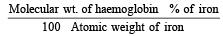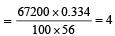QUESTION: 20

In the reaction 4 NH3 (g) + 5O2(g) → 4NO(g) + 6H2O(l) When 1 mole of ammonia and 1 mole of O2 are made to react to completion, 

Solution:

According to Stoichiometry they should react  as follow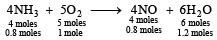Thus for 1 mole of O2 only 0.8 mole of NH3 is consumed. Hence O2 is consumed completely.

QUESTION: 21

An organic compound containing C, H and O gave on analysis C – 40% and H – 6.66%. Its empirical formula would be [1999, 94]

Solution: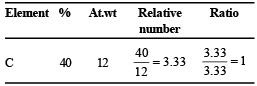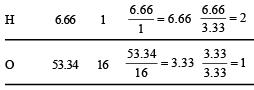(% of O in organic compound Table for empirical formula : = 100 – (40 + 6.66 ) = 53.34 % )
Empirical formula of organic compound = CH2O.

QUESTION: 22

Assuming fully decomposed, the volume of CO2 released at STP on heating 9.85 g of BaCO3 (Atomic mass, Ba = 137) will be 

Solution:

BaCO3 → BaO + CO
197 gm
197 gm of BaCO3 released carbon dioxide = 22.4 litre at STP
∴ 1 gm of BaCO3 released carbon dioxide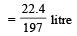∴ 9.85 gm of BaCO3 released carbon dioxide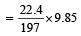= 1.12 litre

QUESTION: 23

Specific volume of cylindrical virus particle is 6.02 × 10–2 cc/gm. whose radius and length 7 Å & 10 Å respectively. If NA = 6.02 × 1023, find molecular weight of virus 

Solution:

Specific volume (volume of 1 gm) of cylindrical virus particle = 6.02 × 10–2 cc/gm
Radius of virus (r) = 7 Å = 7 × 10–8 cm
Length of virus = 10 × 10–8 cm
Volume of virus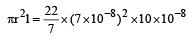= 154 × 10–23 cc

Wt. of one virus particle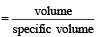∴ Mol. wt. of virus = Wt. of NA particle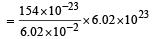= 15400 g/mol = 15.4 kg/mole

QUESTION: 24

Percentage of Se in per oxidase anhydrase enzyme is 0.5% by weight (at. wt. = 78.4) then minimum molecular weight of peroxidase anhydrase enzyme is 

Solution:

Suppose the mol. wt. of enzyme = x
Given 100g of enzyme wt of Se = 0.5 gm

∴  In xg of enzyme wt. of Se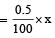Hence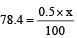∴ x = 15680 = 1.568 × 104

QUESTION: 25

Which has maximum number of molecules?

Solution:

2g of H2 means on e mole of H2, hence contains 6.023 × 1023 molecules. Others have less than one mole, so have less no. of molecules.